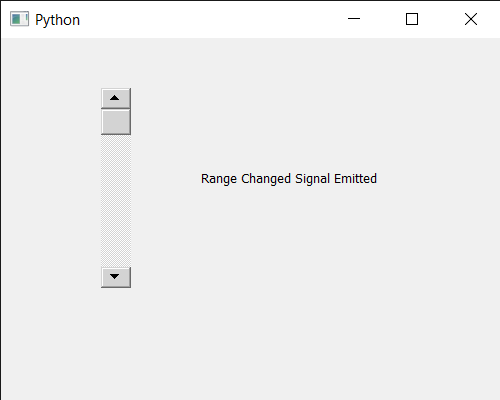GFG App
Open AppBrowser
Continue

# PyQt5 QScrollBar – Getting Range Changed Signal

In this article we will see how we can get the range changed signal of QScrollBar. QScrollBar is a control that enables the user to access parts of a document that is larger than the widget used to display it. Slider is the scroll-able object inside the bar. By default value range from 0 to 99. Value is basically depend upon the slider position, scroll bar value changes according to the position of the slider, value increases when slider goes down and decreases when slider moves up. It can be programmatically set with the help of setValue method. Range is basically a combination of minimum and maximum value which can be set with the help of setRange method. This signal is emitted when the slider range has changed, with min being the new minimum, and max being the new maximum.

In order to do this we will use rangeChanged method with the scroll bar object. Syntax : scroll.rangeChanged.connect(method) Argument : It takes method as argument Return : It returns None

Below is the implementation

## Python3

 `# importing libraries` `from` `PyQt5.QtWidgets ``import` `*` `from` `PyQt5 ``import` `QtCore, QtGui` `from` `PyQt5.QtGui ``import` `*` `from` `PyQt5.QtCore ``import` `*` `import` `sys`     `class` `Window(QMainWindow):`   `    ``def` `__init__(``self``):` `        ``super``().__init__()`   `        ``# setting title` `        ``self``.setWindowTitle("Python ")`   `        ``# setting geometry` `        ``self``.setGeometry(``100``, ``100``, ``500``, ``400``)`   `        ``# calling method` `        ``self``.UiComponents()`   `        ``# showing all the widgets` `        ``self``.show()`       `    ``# method for components` `    ``def` `UiComponents(``self``):`   `        ``scroll ``=` `QScrollBar(``self``)`   `        ``# setting geometry of the scroll bar` `        ``scroll.setGeometry(``100``, ``50``, ``30``, ``200``)`   `        ``# making its background color to green` `        ``scroll.setStyleSheet("background : lightgrey;")`   `        ``# creating a label` `        ``label ``=` `QLabel("GeeksforGeeks", ``self``)`   `        ``# setting geometry to the label` `        ``label.setGeometry(``200``, ``100``, ``300``, ``80``)`   `        ``# making label multi line` `        ``label.setWordWrap(``True``)`   `        ``# getting range changed signal` `        ``scroll.rangeChanged.connect(``lambda``: do_action())`   `        ``# method called when signal is emitted` `        ``def` `do_action():` `            `  `            ``# setting text to the label` `            ``label.setText("``Range` `Changed Signal Emitted")` `            `    `        ``# setting range of scroll bar` `        ``scroll.setRange(``200``, ``250``)`           `# create pyqt5 app` `App ``=` `QApplication(sys.argv)`   `# create the instance of our Window` `window ``=` `Window()`   `# start the app` `sys.exit(App.``exec``())`

Output :My Personal Notes arrow_drop_up